Select Page

# Understanding Time

### Divine Compensation and Understanding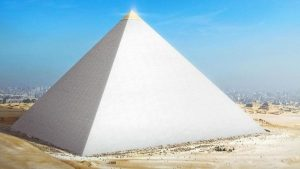In this final section, we will connect the physical with the spiritual as was always intended. The Holy Sparks we were charged to raise were not sitting like glowing pearls upon flower petals in beautiful gardens. They were embedded in stone and granite and needed to be dug out with the sweat of our brow and spiritual equity.

All creatures build homes for themselves, even massive ones. Some build fortresses to defend themselves. Some have graveyards to bury their dead. Humans also build entertainment complexes, libraries, centers of learning or training, manufacturing and warehouse facilities, vehicles and even statues.  None waste enormous resources on pointless monuments, yet there are gigantic pyramids of unknown origin and timeless dating all over the world from the Americas to the Pacific Islands to China, Eastern Europe, Antarctica, and of course Egypt.  Imagining that any of them were designed and built by Egyptian Pharaohs is a fairytale to sell tourist packages.  Those ancient Egyptian kingdoms capable of building fortresses, temples, and palaces did so and with a very particular architecture literally covered in hieroglyphs and decorative artwork. They were beautiful and practical and bear no resemblance to the hundreds of pyramids around the world, which are all stark, void of any markings whatsoever, highly impractical, extremely difficult to navigate, and seemingly useless to any known society in the last 6000 years. Yet even the distant more ancient, fabled ones to which the ancient Egyptians attributed the Pyramids to would not have committed their time and resources to producing useless monuments.  We can only deduce that they had a purpose, an important functional one, one that may or may not be dormant. We also know that all those pyramids were built to last, unlike nearly every other structure known to man.

Most of the self-proclaimed pyramid scholars or worse pyramid debunkers anchor their ideations solely in the stone-cold physical realm. They come up with ludicrous theories that because the Great Pyramid is found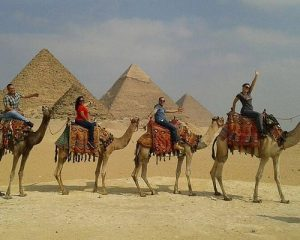in a necropolis it must be a Tomb, completely oblivious to the reality that the necropolis was built around the Pyramid thousands of years later, and that the Pharaohs and Kings Tombs were hidden buried underground miles away, not in the middle of a known cemetery. They might as well call it a barn because camel rides can be had there. They would have us believe that the Pyramid and all other pyramids in Egypt and for that matter, around the world, are empty and devoid of decorations and engravings because they were looted and then the robbers sealed the tombs up after themselves. They would have us believe as they do that the numbers cannot cosmically line up because there is no way G-d or anyone He Divinely inspired could know such things, basically because they do not believe in G-d, or even hyperspace.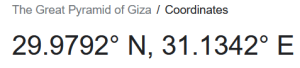For example, the 29.9792458o latitude meridian passing through Pyramid at a point other than its central axis is proof to them that the speed of light at exactly 299,792,458 m/s is just a coincidence and that there is no way for the Creator to know what the speed-of-light is or what the meter would eventually be or that He could not have a purpose for not aligning it through the center of the Pyramid.  This is not even an important tenant of our discussion as we have shown the incorporation of the speed-of-light in miles per second into the Spherical Time paradigm and other significant Cosmic equations including the ratio of Spiritual Time to Physical Time. It does though illustrate how those with their feet in concrete will try to misdirect the tens of millions of the more open souls who have seen videos about the Pyramid’s relationship to the spiritual and esoteric realms. Moreover, as we are about to see, even the misalignment of the Pyramid plays an important role in its design and integration with the Torah and the Cosmos to a fraction of an inch.

Furthermore, they believe and argue that all alignments are coincidental and make excuses that other things in the world align too, oblivious that those align for the very same reason. They believe that anything physical must have been created by Man, including the Torah. They believe everything is set in stone. Unfortunately, Man’s adversaries know better. Fortunately, some of us do too.  The goal is enlightenment.

As discussed, the base measurements of the Great Pyramid are purposely slightly skewed off center and out of alignment with the Cosmic Sun Pyramid by a total of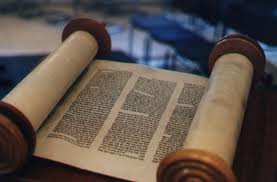24.8 inches or 0.0684%. Those 24.8” is the square root of the value of H’Torah (התורה) and it represents the 248,000φ words, letters, verses, rows, and columns in the Torah. The average per side that they are off from the idealized square base is 6.20” as Keter (620) and the 62 Yuds (י) in the 10 Commandments. As we know, the Hebrew word for 20, as in the 20 stages of the Tower of Truth is 20, esrim (עשרים) has a numerical value of 620. The sum of the factors for the number 20 is (20 + 10 + 5 + 4 + 2 + 1) = 42.  Therefore, the Pyramid’s skewing is aligned with the 42 rows and 248 columns in the Torah. Moreover, there are 223 rows and columns in the Torah, as in the 22nd cube of the 22 cube tower that fits in precisely from the top of the Pyramid to its Subterranean Chamber.

The 62 Yuds (י) in the 10 Commandments are akin to the edge of the Earth’s atmosphere, the Kármán line, at 62 miles, the boundary between our atmosphere and outer space.

Moreover, the Tower and Pyramid reaching upward through 20 stages as in 620, Keter, is akin to the Earth’s final layer, the exosphere that stretches up to 6200 miles, its final limit beyond which there is a vacuum. This in turn is akin to the numerical value of “The Altar (המזבח), 62, found 100 times in the Torah, giving it a total value of 6200. It is also the sum of the cumulative values of the 22 letters, taken one by one: (1 + 3 + 6 + 10…) = 6200. It is also like the 112 initials of the 112 Essential Triplets whose value along with the Primal Frequency as a kolel is also (5925 + 275). The 224 back letters of the 112 Essential Triplets have a value of 11,040 or 40 x (275 + 1).

Meanwhile, the ordinal value of the Altar “The Altar (המזבח), 35, may be akin to the 35th Course and the since (100 x 35) = 3500, it may also align with the 3500 value of the 5 final letters (ךםןףץ) letters. We only suggest this because the Mesosphere ends to 50 miles, as in the 50th Course linked to the 35th through the Queen’s and King’s Chamber dynamic.  Nothing about any of this is coincidental. As we recently saw, the full height of the Pyramid System including the Subterranean portion is 22 stacked cubes and we just saw they are perfectly aligned with the 22 letters and Keter, 6200. This is the Tower that stretches to the furthest reach of our existence, the 6200 miles to outer space.

If we were to add those supposedly misaligned 24.8” to the height of the Pyramid in inches we would get a total of 5800”, as in the Torah’s total gematria sofit of 5800.0542; the 58 words, letters and verses in the Torah, and the Primal frequency of the Planck’s Diameter (27.5 Hz x 21.09143628 x 10-35) = 580.0145…. The addition of 5800 and 6200 is 12,000. It is time we understood.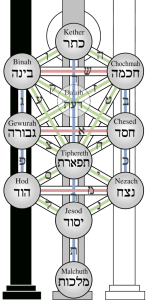Alternatively, if we were to add those 24.8 units as feet to the height of the Pyramid, we would get a total of (24.8 + 481.2) = 506.0, as in the 506 value of the first row of the 42-Letter Name Matrix, which is also the complete value of Moshiach Ben David, the fuller Cosmic Consciousness. Moreover, as the sum first 11 integer squares (12 + 22 + 32112) = 506, it represents the expansion into the 42-letters of the 11 sefirot/dimensions. Thus, the designed and implied compensation of 24.8” elevates the Pyramid’s resonance beyond all the 10-dimensional integrations of the Great Pyramid.  We have highlighted numerous of these multiple of 10 integrations, but they start with its 210 cubit and 210 course height that references the 210 or 10-dimensional hypercube, which itself is aligned with the 210 or 1024 word-values in the Torah.

Is there an implication that the 11th sefirot or dimension represents an expansion into the 248 dimensions as in the E8 Lattice that we have frequently discussed.

Because we could not determine the true value of the Pyramid’s base dimensions without digging up the paving stones, nor determine the measurements of its inner chambers and passage system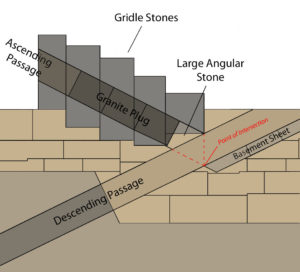within knowing its secret entrance and blasting through several thick granite plugs, might we surmise that we were not meant to discover the secrets revealed by these measurements?

Might it be that whatever purpose the Great Pyramid is serving in the Grand Design of the Creator it is doing so whether we measure it or not. This may be more profound than at first glance because it means that the Great Pyramid might be doing something and interacting with our universe and the Cosmos just by being there in the location chosen for it. After all, knowing the Sun-Earth-Moon ratios and relationships and their—along with the other planets—relationship to the dynamics of Spherical Time and the Phi (φ) field and the Cosmic Harmonic 1.273 does not change the fact that they are continuously interacting with us, the universe, and the Cosmos, or that they have cycles that continually tick whether we are watching the clock or not.  We are here not to alter the Cosmos, but to resonate with them and in so doing learn how to elevate and reintegrate our consciousness.

For a while, people adjusted and expanded the measure of an inch, even inventing the concept of a pyramid inch in order to recalibrate the perimeter of the Pyramid so that it would equal 365.24 days in a year, as if it were a big sundial.  Yet that already happens with just knowing that the Pyramid is purposely off by (365.2436,275/100) = 24.88”, as in the 4 word-values found exactly 42 times in the Torah that add up to 2488, and as in the total volume 7.24888 of the Great Pyramid. And since this equation relates to years, the year 2488 HC is when Moses passed away after 120 years, and when the Israelites entered Israel after (40 + 210) years.  The year 2488 HC is the year 1273 BCE in the Western Calendar, as in the Cosmic Harmonic 1.273 that squares the circle and that defines the dimensions of our solar system and so much more. And all this happened and happens whether we know that there are exactly 248,000φ or 401273 words, letters, verses, rows, and columns in the Torah.

### Casing Stones and Ultimate CapstoneThe Pyramid was originally covered in beautiful white Tura limestone casing stones from the quarries near Goshen. All calculations as to the Pyramids’ size took them into account and were extrapolated from the few stones that survived and the indentations in the corner sockets.

As previously explained, at the base level the casing stones add exactly 466.6” to the underlying dimensions of the Pyramid, or an exact average of 4.241818 cubitsper side/face, as in Moshiach Ben David, the Cosmic Consciousness. The empty sockets extended a few varying inches in each corner.  It would be hard to say that this was not a purposeful design because the diagonals of the pyramid from corner to corner are each 466.6 cubits, which is also the sum of the 11th, 12th, and 13th Fibonacci Numbers (89 + 144 + 233) = 466.

Individually the casing stones added on average 58.3” in each of 8 directions or 116.65” per side of the Pyramid at the base level, or exactly 4.24182 cubits, matching the width of the Antechamber to the King’s Chamber within the 27.5’ long King’s Passage. Those 116.65” are perfectly aligned with 11.66% of the full circle (360o), which is exactly 42o, the edge angle of the Pyramid.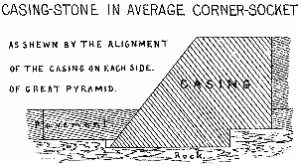Part of that base is beneath the paving stone platform and set into the sockets for design and stability reasons. The above ground or visible portion is exactly 67” as in Binah (67) per side, 33.5” per face. Thus, metaphysically the Pyramid is—was originally—coated in a sheen of Binah and Cosmic Consciousness. And each stone at 2.120 cubits is equal to “The Light, H’Ohr (212)”, as in the first thing G-d saw in our world, “And God saw the light, that it was good” from Genesis 1:4.

It is a shame Man saw fit to remove all those magnificent casing stones.

Per side, the underground or invisible portion is on average 49.65” or (50 – .35) inches, as in the 35th and 50th courses of the Queen and King’s Chamber dynamic, and as in the famous 50 inch 35th course that marks the Great Interior Triangles of the Pyramid. Metaphysically, it may refer to the 50th Gate of Binah, or conversely the 50th gate of negativity.

Moreover, it is fitting that the underground portion is 49.65” since 496 is the numerical value of the lowest sefira/dimension, the one without light of its own, our dimension, Malchut, the screen of the cosmic projection.

The difference between the visible and underground or invisible portion of the base level casing stones is (58.333.5) = 24.8” which is exactly the same 24.8” that the Pyramid at the base (36,275.2”) is off from the idealized 36,300” base of the metaphysical Sun Pyramid, with all the Torah and Cosmic connections to 248, not to mention the Pyramid’s own connections to it and to the 248 dimensions of the E8 Lattice.

Since the visible casing stones add 33.5” inches to each of the 4 corners, or 67” per side, when they came to together at the 210 course per side the ultimate pyramidion or capstone would measure (67” x 67”) and be 4.2426” high, or most probably 66.66” by 42.426” in alignment with the location of the Temple Mount in Jerusalem and the Temple altitude of 2448’ given that we are at 66.66 jubilee years since 2448 HC, in alignment with the Spherical Time Event Horizon.

# 66.60’358 6o

This obviously aligns the Ultimate Pyramidion atop the Pyramid with the entire Pyramid and with Moshiach Ben David, the Cosmic Consciousness that surrounds it based on its casing stones. It also aligns it with the square root of 18.

### The Diagonals and the Names of the Creator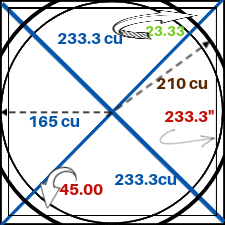The casing stones extend the length of each face of the Pyramid by 116.65” or 233.3” along both axis together.  As we have previously studied, this is aligned with the 233.3 cu measurement of the semi-diagonals that stretch from the Pyramid’s casing stone corners to the central vertical axis of the Pyramid—the Capstone-Great Step- Queen’s Crown axis.

We have previously studied how the 4 diagonals extend 23.33 cu past a circle constructed out of a radius of 210 cubits inscribed within the Pyramid’s base, as in (233.3210) = 23.3 cu.

And since we are speaking about the Pyramid’s corners whose edge angle is 42o, we should point out that 42o is 233.33π radians, and once again the Pyramid aligns with the Singularity of 42, with all 4 corners focused on it in multiple ways.

All three measures within the Pyramid’s square base, 23.34 cu, 233.3”, and 233.3 cu are thus aligned with the second iterations of the Names YHVH (יוד־הי־ויו־הי) and Ehyeh (אלף־הי־יוד־הי) that equal (72 + 161) = 233, the 13th Fibonacci Number.

Because the first iteration of the YHVH (יהוה) and Ehyeh (אהיה) equal (26 + 21) = 47, the two iterations together equal (47 + 233) = 280, as in the 5 final letters (ךםןףץ) and the 280 feet of the full Ascending Passage and hypotenuse of the Great Triangle. It also aligns with the 280-day human gestation period.

And as previously explained, all 3 iterations that total 3142 align with Pi (π); the 42-Letter Name; the powerful Name of G-d told to Moses in Exodus 3:14, “I am that I am (אהיה־אשר־אהיה); the scored lines in the Entrance Passage of the Pyramid, and the perimeter of the central vertical plane of the Pyramid in cubits, (267 + 267 + 330)/27.5 = 314.2 cu. Why so much redundancy in the Torah and the Pyramid alignments? Because it reinforces them and makes for a more powerful antenna or broadcast, strengthening the pathways between our world and the world of infinite consciousness and potential.

There are 42 Letters in the 3 iterations of the YHVH (יהוה) and 41 in those of the Ehyeh (אהיה) or 83 in total, as in the (280 + 83) Days in the 363 Day Cosmic Wheel.

And like the Ultimate Capstone, .3142 radians equal 18o, as in the (524800542774) = (18,00026) difference in the two aspects of the Torah’s word value network, and the 183 verses in the Torah. The concept of 18 is one of the powerful redundant core alignments integral to the Torah, Pyramid, Spherical Time, and Cosmos.  Its role is complementary to 72 and their angles form 90o.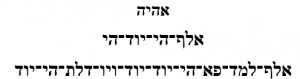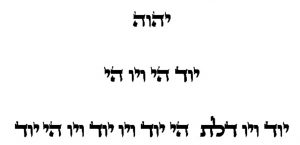As the semi-side of the Pyramid’s base is 165 cu, it is thus equal to the radius of the inscribed circle of the Pyramid. A circle formed using the height of the Tower 210 cu would be (210165) = 45 cubits wider than the inscribed circle and the 4 diagonals would extend 23.33 cu past it in alignment with the 233.3” casing stones and the 233.3 cu semi-diagonals. And just as the 233.3 base measurements align with the Names of G-d, the 45 cu difference in the two radii aligns with the Aspect of the YHVH mah (יהוה) associated with Man (יוד־הא־ואו־הא), as if one is a fuller/richer spiritual energy network than the other.

As for all those 233.3 measures set into the base of the Pyramid, they all equal 233.3 = (232 + 1.3) or His Name (232) and One (13). The value 232 stands for all 4 Aspects of the YHVH (יהוה) and its total value with the kolel for the 39 letters and 4 Names is 275: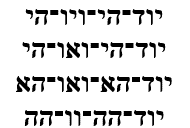Subtracting from the 4 semi-diagonals of 233.3 cu each, their 165 cu counterparts or the semi-sides or semi-diameters of the Pyramid’s base, equals 4 x (233.3165) = 273, as in Absolute Zero or –273o C (0 K); the cosmic microwave background (CMB) radiation in the universe (2.73 K), the temperature of nothingness; the core temperature of the Sun (27.3 million degrees F); the sunspots revolutionary period (27.3 days); the lunar orbit (27.3 days); and the ratio of the Moon to the Earth and Moon together, the Cosmic Harmonic.

Constructing a square out of the midpoint in those four 165 cu semi-sides or semi-diameters of the Pyramid’s base gives us a central square within the Pyramid’s base that is half the area of the base or precisely 72 squares of 27.5 by 27.5, making the full base of the Pyramid equal to 144 squares of 27.52 or a perfectly square grid of 122 x 27.52. This matches the 14.4% ratio of the Pyramid’s slope angle (51.84o) or 722 to a full circle (360o), or (51.84/360) = 14.4. The resonance between the Core Concept of 72 and the Primal Frequency (27.5 Hz) rings throughout the Pyramid, especially concerning its base.

### The Apothem and Jacob’s Ladder.

As explained previously there is a ladder, Jacob’s ladder, that rises along the 7 tiers of the 42-Letter Name Matrix. Each of the 7 rungs of this metaphysical ladder consist of the alternating pairs of the Names Ehyeh (אהיה) and YHVH (יהוה). It is the ladder through Zeir Anpin to Binah. The collective value of the 7 Names Ehyeh (אהיה) is (7 x 21) = 147, the number of years Jacob lived, and the value of the 7 Names YHVH (יהוה) is (7 x 26) = 182, numerical value of Jacob. Together they represent the 14 Names that support Zeir Anpin in the center of the Magical Essential Cube of Creation and align with the 14 Triplets of the 42-Letter Name.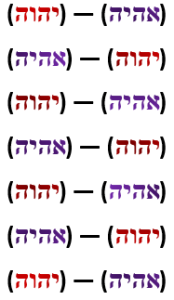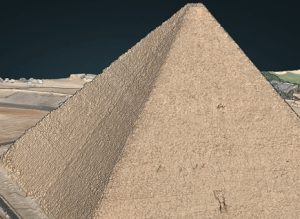The product of the two sums of the 7 Names is (182 x 147) = 26754, as in the Pyramid’s 267 cu Apothem that is scored into each of the 4 faces of the Pyramid; and as in the 54 portions and cycles in 5778 and of the Torah; and as in the 267th Triangular Number, 35778; and as in the average base of the Pyramid (755.8’) that is 1/2.67 of 2018 CE, the Western analogue to 5778 HC, and the 2018 year radius of Physical Time.

The 267 cu Apothem is also aligned with the 26705 sum of the 90 doubled words (or 180 in total) that represent the linkage between Zeir Anpin and Binah in the Torah, which in turn matches perfectly the 267.050 square root of the total ordinal value of all the letters Zadi (צ) in the Torah, whose numerical value is 90, and whose ordinal value is 18.

The Pyramid’s Apothem is 612 ft, or 22.25 cycles of 27.5 Hz, making it the Phi (φ) angle complement (222.5o) to the 137.5o Phi (φ) angle at the core of the 360o Spherical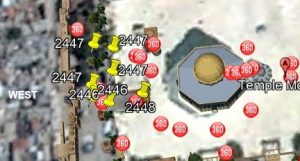Time. Moreover, the 4 Apothem slant heights that are scored into the 4 faces of the Pyramid have a total distance of (4 x 612) = 2448 feet, as in both the year (2448 HC) of the Exodus and that the Torah was received at Mt Sinai and in the elevation of the Holy of Holies on the Western edge of the Temple Mount in Jerusalem, 2448’.

### The Numbered Paths

The total value of both sets of spelled out Names Ehyeh (אהיה) and YHVH (יהוה), (232 + 455) plus their 69 letters equals 756, the base measure of the Pyramid, 756 feet, matching the ordinal value of the two corresponding Magical Essential Cubes of 42, that of Binah and that of Zeir Anpin, (378 + 378) = 756.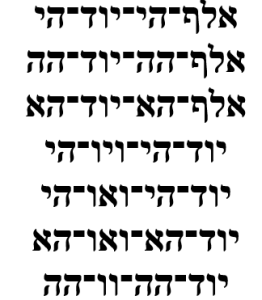The 455-value of the 3 Aspects of Ehyeh (אהיה) of Binah is in the all-important Path of One (1 – 13 – 91 – 455 – 1820).  The Number Paths are the ascending values of their cumulated summations, going from simple sums to Triangular Numbers to Tetra Numbers, etc. They are easily recognizable when organized into Pascals cascading Pyramid, where we see the parallel Paths of 5, 9 and 13. Organized this way, the Paths naturally intersect, and many of our core numbers appear in multiple paths, which only emphasizes their importance to the dynamic networking of the Cosmos. Of note about the Path of 5 (1 – 5 – 15 – 35 – 70 – 126 – 210330 – 495 – 715 – 1001 – 1365 – 1820) is that it not only includes the important core Pyramid values, 35, 210, and 330, the 495 and Phi (φ) circle but its 13th member is 1820 from the Path of One (13) and the 1820 YHVH (יהוה)  in the Torah.

Of note about the Path of 9 (1 – 9 – 45165495 – 1287 – 3003) is that it not only includes the 45, and the 165 and 495, but the 3003, from the sum of the 11 Triplets of Bereshit.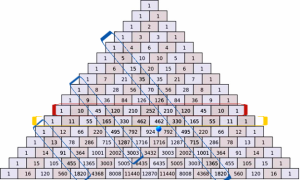While the Pyramid numbers crisscross Pascal’s pyramid, we should not that the design of his pyramid is such that each row total’s 2n, as in the 10th row that contains 210 totals 210, as in the 210 word values in the Torah.

That so many of the Great Pyramid’s core numbers are found in Pascal’s Pyramid can not be coincidental as Pascal’s cumulative numbers are far rarer than might appear. Excluding the integer row from our calculations for obvious reasons, there are only 64 cumulative numbers through the number 1000. Dynamically, there are only 10 through the number 55, and only 26 through the number 210, leaving exactly 55 of them starting from the number 55 through 1000, yet another reason 55’ was chosen to be the entrance height of the Pyramid.  Nevertheless, of those 64 cumulative numbers, 42 of them are Triangular (3rd order) Numbers, leaving 22 as complex cumulative numbers, as in the 22 letters, or fields, of the Alef-bet.

In the center of the Pyramid we see numerically translated the expansion and progression of the YHVH (יהוה) and its component letters from Yud (י) to Yud (יוד) and Hei (ה) to Hei (הי) to Hei Yud (יוד)־(הי) to the 2 Hei Yuds (יוד)־(הי)־(יוד)־(הי) in the 3rd iteration of the Name.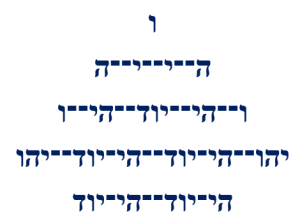The 3 iterations of the Letter Hei (ה) is 55, as in the entrance gateway, the ordinal value of the 3 iterations of the Name Yah (יה) is 165, as in the semi-base.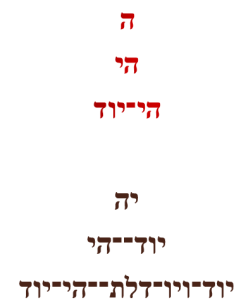### The 444 Years of Dust and Darkness

The combined ordinal values of the 7 Names (232 + 212) = 444, which is like the 156th course of the Pyramid that is 4444 inches high, as in the numerical value of Joseph that is (6 x 26) = 156. This is like the first and second plagues in Egypt whose gematria values are 44 and 444 respectively.

Thus, the total complete value of the 7 expanded Names that can help us bridge the spiritual gap through the 7 dimensions and ladder of Zeir Anpin that is the engine of physicality to Binah, the level of consciousness, is (756 + 444) = 1200, and thus fully aligned with the Time Constant (12),

The oft quoted Kabbalistic phrase “He and His Name are One,” comes from Zechariah 14:9.

# וּשְׁמוֹ אֶחָד. בַּיּוֹם הַהוּא, יִהְיֶה יְהוָה אֶחָד

While the 7 final letters of “On that day shall He and His Name be One,“ add up to 625, as in H’keter, the rest of the letters, or the front letters in the verse equal 444, which is also the gematria value of the important Torah phrase “from generation to generation (לדר־ודר)” as in Rav Ashlag’s equation 444/22 letters = 20.18, like the year 2018 CE, the Western analogue to 5778 HC.  When Rav Ashlag calculated the timing of the Event Horizon as 5778 HC, he used the .333/.666 proportion in 3 iterations starting with the prophesied 6000-year period that was based on the 6 Days of Creation. Going from a first (2000 + 4000) = 6000-year split to a second (666 + 1333) = 2000 year one, the final 666 years were split into 222 and 444 years, hence (6000222) = 5778. As it happens, the 42” x 127” dimensions of the Queen’s niche have an area of (42 x 127) = 5334 or (5778444).

Rav Ashlag did not know this by 666 using the Prime Number Theorem we see that both 5775/ln (5775) and 5778/ln (5778) is approximately 666.

### The Clock Face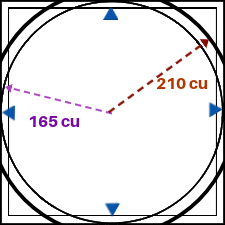If we use the Pyramid’s height as the 210 cu radius of a circle it would be as if the circle was drawn vertically around a mirrored Pyramid, in other words with the central Tower of Truth as its radius.  And viewing either the virtual vertical circle or the flat base circle as a clock face, the 210 cu Tower would be the minute hand. The shorter minute hand could be the 165 cu radius of the circle inscribed in the Pyramid’s base.

The Face of the Clock would thus be a circle drawn around the outstretched hour hand with a diameter of 420 cu, giving it a circumference of 420π and an area of 2102π.  Its circumference matches the 1320 cu perimeter of the Great Pyramid that inscribes the minute hand circle, and thus every hour would sweep through (1320/12) = 110 cubits, as in the first interval of the Primal Frequencies.

One hour of the 420π circumference of the Clockface would be 420π/12 = 35π, as in the doubled 35th course that marks the height of the Queen’s Chamber and its dynamic with the King’s Chamber, and 35π = 110, as in the major interval in the Primal Frequency, the Interval of Miracles.  All 12 hours or (110 x 12) = 1320 cu, the perimeter of the Pyramid, making a cycle of 12 intervals, like the 12,000 year periodicity of the Galactic Current.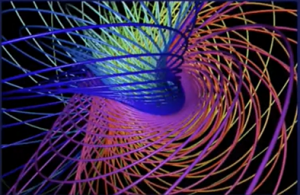The minute hand would be based on the cosmic clock and like the Hebrew clock there are 18 parts to every minute, so every hour contains (60 x 18) = 1080 parts of a minute, or twice 540, the sum of the Pyramid’s height and base (210 + 330) = 540. Therefore, the 330π circumference based minute hand sweeps through (330π/12) = 86.4 cu every hour, as in the diameter of the Sun (864,000 mi) and 330π/(12 x 1080) = .08000 cu every part of a minute. This works out to 2.2 inches or .1833 feet every part of a minutes, like the 22 Letters and the 1833 letters Samech (ס). So maybe the Pyramid is a sundial after all, just a very sophisticated one in tune with the subtleties of time and space, and in harmonic resonance with the Cosmic Wheel and the design of our Solar System and beyond.

The Earth’s 7920-mile diameter can also be seen to be incorporated in the Pyramid’s Clockface in that 7920 = (330 x 24) as in the 24 hours in a Day, and since the Moon’s 2160-mile diameter equals (90 x 24), the Earth and Moon together represent exactly 420 Days of 24 hours. Thus, the Earth and Moon are represented by the two diameters and sweeping hands of the Clockface: The Earth alone as the inner 330 cu one, and the Earth and Moon together as the wider outer 420 cu one.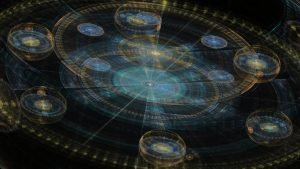Over a 24 hour day, those 1080 parts of a minute equal 25,920, as in the 25,920 year great Precession of the Earth’s axis, and the Cosmic Wheel’s 12 Moons, whose lunar arc matches their 2160 mile diameter.  To be clear, the Pyramid’s Clockface’s minute hand is a normal 12 hour clock with each hour being 1080 parts of a minute or half (2160/2) the lunar diameter, which is the lunar radius. So, we can see that the ancient Hebrew time system was based on the lunar radius thousands of years before they could calculate that radius.

The Architect of the Pyramid and our universe would have obviously known all about the size and orbits of the planets.  He would have certainly known that the planet Saturn completes one precessional Great Year of 25,920 earth years, about every 864 of its years exactly as the minute hand of the Great Pyramid; and that Saturn’s day is 10.7 hours, as in the 107th Triangular Number, the 5778 year radius of Spherical Time or Event Horizon, and the 107 AU radius of the entire planetary system; and that Saturn’s year is equivalent to 10,756 Earth days, as in the 107th Triangular Number again and the 756’ base of the Pyramid; and that Saturn’s orbital period in Earth years aligns with the Moon’s 29.5 day lunar month; and that Saturn’s 36,184 mile radius is only off from the Pyramid’s idealized 36,300” perimeter by 116, as in the King’s Antechamber 116” length, and off from the actual measure, 36,275” by 91 inches.

As for the 233.3” casing stones, the 233.3 cu semi-diagonals and the (233.33210) = 23.33 cu difference of the diagonals and the outer Clock Face, the inner Clock Face in parts divided by 233.3 equals (12 x 1080)/233.3 = 55.550, as in the 55’ entrance height to the Pyramid, etc.

And just like 330π/(12 x 1080) = .08000 cubits for every part of a minute, that same circle of parts divided by Phi (φ), equals (12 x 1080)/1618 = 8.00.

The (1080 parts x 12 hours) in the full Clock Face divided by Pi (π) and/or the Names of G-d (3142) equals (1080 x 12)/3142 = 4125 and 4125” is equivalent to 150 cu, which reflects the 150 Lights of Abba drawn down into Zeir Anpin on Rosh Hashannah as explained by the Arizal. It is also the (10 x 412), the value of the letter Bet (בית), which is the letter Abraham Avinu assigned to Saturn 3800 years ago. Meanwhile, the (1080 parts x 12 hours) in the Clock Face equals (150 x 86.4), as in the the Sun’s diameter once again, and the 3 turns of the 137.5o Phi (φ) angle equals that advanced the Spherical Time pathways, equals 412.5o.  This is like the 4 sets of the 112 Essential Triplets whose average is (16965/4) = 4241.25.

While the Pyramid’s base less those 4125” is (90754125) = 4950, that same inner minute hand’s parts for a full 12-hour sweep divided by the same Phi (φ)2 angle that all the inner passages and Triangles are based on is (12 x 1080)/2.618 = 495.0. Once again, all aspects of the Pyramid work together harmonically with the Pi (π), Phi (φ), the Names of G-d, our Solar System, Spherical Time, and the Cosmos.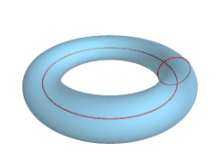The generation of the Spherical Time radial time-paths comes from the spinning of a central Phi (φ) vortex upon a naturally drawn or virtual circle that grows organically into a sphere and eventually a torus. It is the property of Phi (φ) that no two spiraled lines can ever cross that circle at the same point. This is what makes each time-path unique, and it allows for infinite generation without chaos. Each new time path is etched with every 137.5o turn of the Phi (φ) vortex upon the circle. Thus, in a single day of our time (25,920 years x 365.256 days x 24 hours x 60 minutes x 18 parts of a minute), 10,224,830,362 different time paths are generated.  That is with the Spherical Time clock turning once every 3.333 cosmic seconds, or 495o in 10 cosmic seconds. That rotation of 495o is equivalent to the sum of the values in the natural split of the first 18 letters of the Alef-bet from Alef (א) to Zadi (צ); the sum of the 3 x 3, 6 x 6, and 9 x 9 normal Magic Squares (15 + 111 + 369) = 495; and to the sum of the 10 residues of the frequency of 27.5 Hz Quotient: (9, 18, 27…90).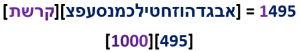While the 165 cu minute hand or radius of the inner Clock Face represents the 165 small gematria0 value of the 42-Letter Name it is also exactly 1/3rd of 495.  The other 2/3rd is 330, the diameter of the inner Clock Face, which is the circle inscribed by the Pyramid. It is also (12 x 27.5), as the fingerprints of the Primal Frequency (27.5 Hz) all over the Clock Face.

Moreover, the 495o of rotation is a turn of 1.375% of the 360o circle, as in the Phi (φ) angle, 137.5o related to the Primal Frequency (27.5 Hz).

### The Turning of Time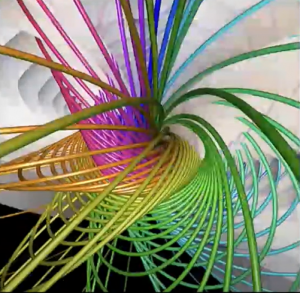That 495o of rotation or turn of 1.375% of the 360o circle advances the hour hand on the circle by 2.75π radians at a time. This is the natural heartbeat or rhythm of the Spherical Time bubble and physicality.  This is so because the Primal Frequency (27.5 Hz) turns the circle at the Phi (φ) angle (137.5o). 2π radians complete 1 circle of 360o and .75π radians represent 135o of a circle or 37.5% It is also .273 of a cubit (2.75) or the Primal Frequency as in the Harmonic Constant (.273). Hence, we see two Primal factors coming together in this dynamic at the core of Spherical Time. We also see the reason behind having a second ¾ length cubit and that the 628” scored line represents the 6.283 or 2π radians that complete 1 circle of 360o, while the other scored line 481” represents 4.81π or 275o.  The Pyramid is a bridge between worlds and no amount of academic nonsense or sensational speculation can tie that to Egyptian technology.

As we studied earlier, the movement of the cosmic clock by increments of 9o in cycles of 10 gives us 10 resultant angles (9 + 18 + 2790) that sum to 495o of rotation. This creates both the Phi (φ) angle at the Source of the infinite paths of Spherical Time and the measure of the cubit (27.5”), which is the resultant 2.75π radians arced around a circle divided by Pi (π). In other words, the cubit is the diameter of a circle formed by the arc of 495o on a circle of radius 1.

We thus see that there are 40 increments of 9o in a 360o circle as in the 40 weeks or 9 months human gestation, and that in the 495o of rotation there are 55 increments, as in the Pyramid’s 55’ entrance height.

When the 495o of rotation is divided by the pulse of the Primal Frequency (27.5), we get 495o/27.5 = 18o, and the sum of their 10 radians for the 10 resultant Quotient angles is Pi (3.14159…).

That extra 135o angle that wraps around the circle is .375 or 37.5% of the circle, leaving a remainder of .625 of the 2nd encircling, as in (2.0001.375) = .625, H’Keter, which is 5/8.

It also matches the standard gematria value of the entire Torah divided by the full sofit value that is 21009826/(58002 + 625) = 21009826/33640626 = .625, approximately 62.5%, and their difference is 12630800/33640626 = .375, approximately 37.5%.

Conceptually before the Torah was Designed and Created there existed the 112 Triplets and before that the Source or Singularity of 42, and 42/112 = .375.

The Singularity of 42 is neither the 42-Letter Name that comprise the 14 Triplets nor the 42 Letters of the Name YHVH (יהוה) that are concealed within the Shema to form the 15 Triplets. These are two of the 4 Essential Elements of the 112 Essential Triplets and are each Aspects or reflections of the Singularity, but not the Singularity itself that exists on a higher plane or dimension.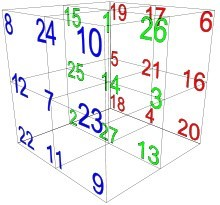If the Triplet (3’s) pattern continued up the hierarchy and the Singularity of 42 were conceptual Triplets of some kind, then we could visualize the (42 x 3) = 126 Primal Elements as projections onto each of the 6 faces of the Magical Essential Cube of 42, and of those 126 Primal Elements, 112 could be projected into the 8 corners of the Cube collectively, leaving (126112) = 14 to be injected into the central position 14 of the Cube. Thus, we have the Creation of the Magical Essential Cube of 42, the Essential Cube of Creation, whose total value is 378 or 1.375 x 275, the Primal Frequency and the full 495o of rotation in a complete turn of 1.375% of the 360o circle of Spherical Time.

### Counting Time

The Cosmos count time in the hierarchy of 12’s the same way we count in feet of 12 inches and as was done in the Pyramid thousands of years ago. The value 25,920 is equal to 12 x 2160, the diameter of the Moon, which is thus equivalent to 120 x 216, the age of Man times the sefira of Gevurah (216), Judgement. There are also 216 letters in the 72 Triplets, while the Great Precession advances 1o every 72 years, in other words 25920/ 360o = 72.  Those values of 216 represent the dimensional folding of space as 216 is a cube or 63 where each edge is the concept of the letter Vav (ו) of numerical value 6, and all 12 edges equal 72 and its surface area like its volume equals 216.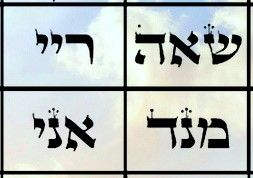It cannot be overlooked that the ratio of 210 to 360 or 210/360 is equivalent to 42/72 or that the 21st letter in the Alef-bet, is the letter Shin (שין) of numerical value 360. Or that at the center of the 4 Triplet summit platform of the 72 Triplet Pyramid—the 28th and 29th and the 36th and 37th Triplets—the central 4 letters (הראד) add up to 210, and the outer 4 letters of the platform (שמיי) add up to 360.

The 210/360 ratio equals .58333 or (1 – .41666), while the average letter value of the 64 Triplets that form the 8000-value square within the 72 Triplets is exactly 41.666. We must always keep in mind that the Triplets exist in a higher referential plane then our limited consciousness can reach and far beyond the processing power of our minds, so any proper connections we can make with them strengthens our ability to access Understanding, and it is only through Understanding that we can reintegrate ourselves into the Cosmic Consciousness. This is why clues were left for us, why the interface language was gifted to us and why the Pyramid exists to this day despite man’s efforts to deface and destroy it.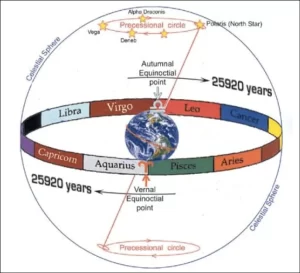Those 72 years can be thought of as a radian in the Great Cycle, and if the Phi (φ)-clock turned its 495o or 1.375% in those 72 years. It would turn 495 rotations over the 25,920-year cycle.  Each of those 495o cycles equals 8.64 or 2.75π radians, and those 8.64 radians align with the 864,000 mi diameter of the Sun, and the hourly sweep of the minute hand in the inner Clock Face, 330π/12= 86.4 cu.

Over that 72-year span or 1o of the circle of the Great Precession, we can divide 72/2.75 = 26.1818 to get approximately 10 Phi (φ)2, as in the Passage angle of both the Ascending and Descending Passages in the Great Pyramid and as in all the dependent interior triangles.

When we then divide that result by Pi (π) we get 26.1818/π = 8.333… or approximately 1/12, representing the universal constant of time (12).

What this means is that the 25,920-year Great Cycle is the measure of 1 unit of the constant 27.5 times Phi (φ)2 for each degree of the circle (cycle), with Phi (φ)2 as 2.61818, representing the Primal relationship of 72/2.75: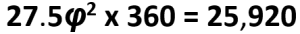The Primal Frequency (27.5 Hz) is space. The cycle is time.  Time is not a dimension. It is an interaction. Space and Time interact.  This is the purpose of the Pyramid. The circle is guided by the Pi (π) field. It is the interaction between the Phi (φ) field and the Pi (π) field that creates the infinite non-chaotic pathways of Spherical Time.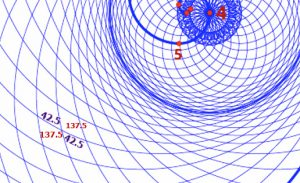The Name YHVH (יהוה) has the numerical value 26 and when spelled-out in its highest Aspect it becomes the more powerful Name YHVH (יוד־הי־ויו־הי) of numerical value 72, so based on what we have just learned the factor of expansion within that Holy Name is the Primal Frequency 27.5. Like the individual letters, the Names of the letters or in this case the Name of the Name is on a higher dimensional level than the Name or individual letters themselves. Thus, this expansion based on the Primal Frequency 27.5 is a dimensional expansion. Alternatively, the Name YHVH (יהוה) is a dimensional contraction from the Name YHVH (יוד־הי־ויו־הי), as in what occurs from going from the dimension of Binah to Zeir Anpin.

Naturally, the ratio of the square base to the inscribed inner Clock Face 3302/1652π = 1.273, the Cosmic Harmonic, but the Pyramid was designed so that the ratio of the area of the outer Clock Face to the square base of the Pyramid would also be 2102π/3302 = 1.272215, or almost exactly 1.273, the Cosmic Harmonic.  Moreover, the ratio 1.272215 is off from the 4/π Cosmic Harmonic by .001024, as in the 1024 or 210 word values in the Torah.

The Clockface and the dynamics do not change whether we use a flat platform base clock or a vertically mirrored hourglass shaped summit-summit pyramid one, but its alignment with the cosmic make up of our universe and hyperspace certainly does.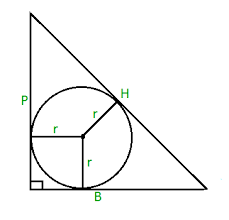Let us go back to the base of the Pyramid, where we see it marked by a crosshairs of 165 to each side and a vertical tower of 210 at its center. Given the angle of ascent and simple geometry the radius of a circle drawn to neatly fit inside that vertical triangle (165210267) is 54, as in the 54 portions of the Torah. When that triangle is part of a 4-sided pyramid such as the Great Pyramid, that circle can be made into a sphere of radius 54 and exactly 4 such kissing spheres can be symmetrically constructed inside the Pyramid. Then, if the circle, or ring, were to be generated as a series of rings pivoting on the central vertical axis, we would have a torus of diameter (54 x 4) = 216 cubits, as in the 216 letters in the 72 Triplets.

Now with our vertical Clockface, there would be 2 stacked tori and their positional dynamic would match both the observed and theoretical shape of the pressure mediations and forces in our universe and in hyperspace.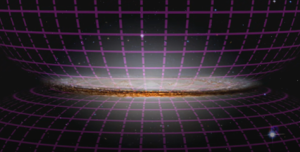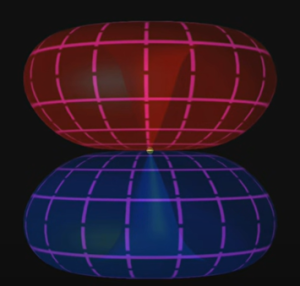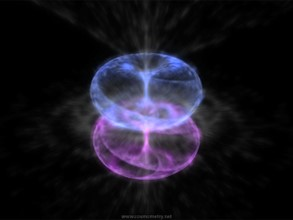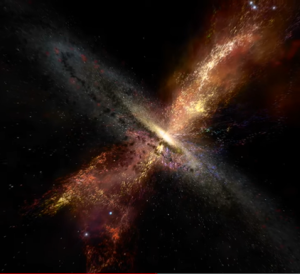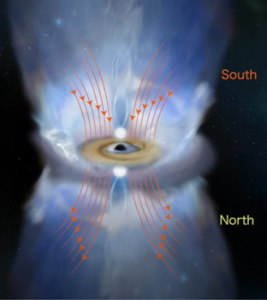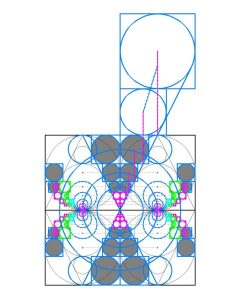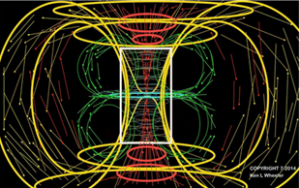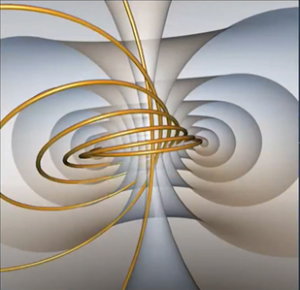# The HourglassThe vertical mirrored summit-summit pyramids may be more of an hourglass than a sundial, with a limited amount of sand. Those 420 years at the pace of the Primal Frequency equal (420 x 27.5) = 11550, the Event Horizon Diameter and the end of the full galactic cycle. They are also the complete value (420) of the word pyramid (פירמיה).

### Our Solar System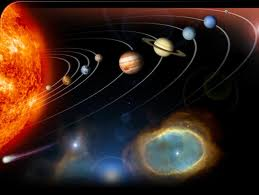The Clockface is integrated into the dynamic design of the Cosmos in myriad ways. One of which is through the surface temperatures of reflective energy levels of our Solar System. The surface temperature of Mars is 210 K; and that of the two neighbors Saturn (134 K) and Uranus (76 K) together is also (134 + 76) = 210 K, making the 3 of them equal to 420 K, or the two Hands of the Outer Clockface; the surface temperature of Jupiter is 165 K, as in the minute hand of the inner Clockface; and Pluto’s is 50 K, as in the 50” of the 35th Course dynamic between the Queen’s Chamber and the King’s Chamber at the 50th Course; while Neptune’s is 72 K, as in the 722 slope angle of the Pyramid etc.; giving all 6 of the planets beyond Earth a combined surface temperature of 708 K, as in the value of the Upper 42-Letters of the Name of YHVH (יהוה), and the value of the 42-Letter verse in the Song of the Sea known as the Sword of Moses, 6708.

Likewise, the surface temperature of the Earth (288 K), combined with those of the four (4) planets flanking Jupiter (210 K + 288 K + 210 K) are also equal to 708 K with Jupiter being the 5th planet from the Sun.  While the first time the Name Moses is found in the Torah is at Exodus 2:10, the Name Moses (משה) is also found within the Hebrew word (חמשה) for the number “5” in the Torah, and this word is found 72 times there, like the 5th Name (מהש) of the 72 Triplets. That value of 708 K is also found in the year Israel became a nation, 5708 HC or 1948 CE, 3760 years after Abraham’s birth in 1948 HC.

With the surface temperature of the two inner planets, Mercury (440 K) and Venus (737 K) at .72 AU from the Sun, the 9 planets have a combined surface temperature of 2173 K, which is .3760 or 37.60% of 5778 K, that of the Sun, just like the 37.60 cumulative AU distances from the Sun to Uranus, out of the total 107 AU distances for all 9 planets, as in the 107th Triangular Number, 5778.

It is also like the 3760 mi radius of Venus, and the Spiritual Time component (3760 years) of the Spherical Time radius (5778 years) that aligns with the pivotal hinge year (3760 HC) of the Gregorian and Hebrew Calendars.

That Uranus has a surface temperature of 76 K is interesting unto itself considering that the 4 core Pyramid numbers add up to (55 + 165 + 330 + 210) = 760, and that 76 is the ordinal value of the first word of the Torah, the square root of 5775”, and the descending angle (76o) of the 4-sided Pyramid. They also perfectly align with the 3 highest sefirot (dimensions) Keter (כתר) and Chochma (חכמה) and Binah (בינה) as (620 + 73 + 67) = 760.

### The TowerThe Time paradox is seen in the Tower of Babel where the one world order was in a rush to reach the top of the Tower back in 1776 HC (- 1984 BCE). The Truth is that when they did,they also reached the bottom of the Tower at the opposite end of Time. At (210 + 210) = 420, it was not an opportunity to get high; their time was up. This happened exactly 120 years after the Flood, the Age of Man, as in the base less the height of the Tower and the Pyramid, (330210) = 120. If that time period seems short, ask what man and his new one world order have built and achieved in only the last 120 years?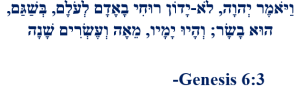The exact 13 words uttered by G-d are “My spirit will not continue to judge man forever, since he is nothing but flesh. His days shall be 120 years,” whose final letters total 444, and the 20 letters in the 5 words “His days shall be 120 years” have an ordinal value of 210, as in the 20-stage height of the Tower and Pyramid. That same height of 5775” divided by the age of man (120) is 48.125, representing the 481.25’ height of the Pyramid, while (210 + 120) = 330, the Pyramid’s base.  Moreover, while the phrase “since he is nothing but flesh” has a sofit value of 2755, as in the Primal Frequency and the 55’ entrance, it also connects with Abraham and the Torah’s first verse, 140 verses earlier, as in Chochma (חכמה) and Binah (בינה) or (73 + 67) = 140.

### From the Beginning

Out of the first 8 words of the Torah that form the 33 Triplets of Creation, one letter in each of the first 5 words spells out Abraham (אברהם), the first Man in the Torah.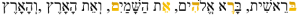Since the gematria of those first 5 words is (666 x 3) or 1998, the net value after separating the 5 letters of Abraham (אברהם) is (1998248) = 1750, or (10 x 175), as in the 175 years of Abraham’s life. What is this like? It is like the 5 Books of Moses whose Names (Bereshit, Shemot, Vayikra, Bamidbar, and Devarim) add up to 2480 or (10 x 248), Abraham. Since 50 is (10 x 5) following that same pattern and because the final 2 letters in the 5 words equal 50 the phrase can be said to indicate that (199850) = 1948, the year (1948 HC) of Abraham’s birth, and that of the State of Israel (1948 CE) as well.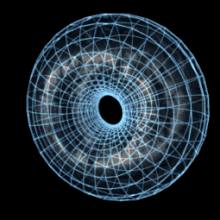While the year 1776 CE coincides with the date that the 13 colonies became a nation, the year 1948 CE coincides with when the remnants of the 12 Tribes became a nation in 5708 HC, and the product of those dates with the Primal Frequency equal (5708 + 1948) x 27.5) = 210540, as in the Pyramid’s height and base plus height measurements. If instead, we subtract the year of the Flood from them, we get (5708 + 1948) – 1656 = 6000, as in the 6th millennium, the common prophetic time span for the final redemption, and the half galactic cycle. Moreover, (5708 + 19481656) x 27.5 = 165,000, as in the 165 cubits of the Clockface minute hand and radius, the midpoint of the Pyramid’s base and Jupiter.

This leads us back to the Pyramid’s apothem, 612’, which is the gematria of Covenant (Brit). When divided by the Primal Frequency 27.5, we get (612/27.5) = 22.25, as in (6000222) = 5778, the Event Horizon. This is like 4 face to face edge angles of the Pyramid plus the two apex angles of its summit that total (4 x 112 + 2 x 76) = 600o.

As for the rest of the 8 words or 33 letters, the net (335) or 28 letters equal (3003248) = 2755, as in “since he is nothing but flesh.”

Since the total value of those 33 letters or 8 words is 3003 of which the 8 final letters equal 1661, the rest of letters must equal (30031661) = 1342, as in the complete value of the Torah’s first 3 words or 14 letters (בראשית־ברא־אלהים) that equals 1342.  While the first of the strings …5778… in Pi is found at digit #633, the full sequence …577134275778… begins at digit #625, as in H’Keter, and includes both 1342 and 275.It is also the ratio of the 72 Triplet Matrix to its summit platform 9143/681 = 13.42584.

Then since the second to last letters in each of the 8 words total 432, as in the frequency of Binah (432 Hz) and radius of the Sun as explained earlier, the other 17 letters equal 910, like in the 17th paragraph of the Torah when Kenan (קינן) lived 910 years, as in 10 x 91, twice 455 and half 1820 from the Path of One.  The numerical value of Kenan (קינן) is 210 and the Torah’s first 17 letters have the complete gematria of 1776.

The 120th year after the nominal year of the Flood (1656 HC) is 1776, the year of the formation of the United 13 Colonies and of the modern-day Illuminati.  While (4 x 444) reflects the Torah phrase “from generation to generation (לדר־ודר),” which Rav Ashlag explained represents 444/22 = 20.18, it is also equal to 1776, the collective perimeter of the 3 concentric walled rings—the Central Altar, the Inner Courtyard, and the Outer Courtyard—of the Future Holy Temple: (32 x 4) + (100 x 4) + (312 x 4) = 1776 cubits.

Moreover, there are exactly 1776” until the 54th course or the Pyramid, leaving 4000 above it.

The same forces that put the Latin words for “New World Order” beneath the 13-tiered 72 block pyramid on the One-dollar bill, also engraved 1776 in 9 Roman numerals (MDC, CLX, XVI) into it, which when divided into triplets have 3 initials that total 1110 and 6 backs that equal 666, like the middle letters in the Biblical separation of Light from darkness.  It is also like the 1110” Entrance Passage of the Great Pyramid.

In the cycle of time, everything repeats itself. It is built into the system. The logarithm of the Event Horizon 5778 is 3.761, or year 1.

### Names in Time and a Prophecy

In Genesis we learn that Cain (קין), whose name is the root letters of Kenan (קינן) got jealous, killed his brother, and went to Nod (נוד), East of Eden.  “The Path of the Tree-of-Life” was East of Eden. Cain built a city that he named Enoch (חנוך).  Generations later Nimrod (נמרוד) built a city and a tower in order to place Malchut on top of Zeir Anpin.

As discussed before, it was not just a Tower but also a city. We hear of a city in Egypt mentioned again in a prophecy of Isaiah.

## -Isaiah 19:18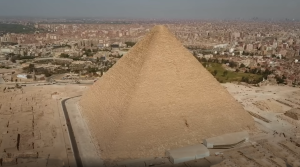Isaiah goes on to say in the next verse, “In that day shall there be an altar to the LORD in the midst of the land of Egypt and a pillar to God at its border.” We cannot say for certain, but “a city and a tower” and a city of (ההר), the mountain, and a pillar seem to be a powerful juxtaposition with a perfect candidate.

All 3 Names, Nod (נוד), Enoch (חנוך), and Nimrod (נמרוד) contain the letters (נו) of ordinal value 20 while Nimrod (נמרוד) contains Nod (נוד) entirely with the addition of (מר), whose numerical value 240 is the same as Amalek (עמלק), the scourge of the Israelites.  Nimrod’s name (נמרוד) also ends in (רוד) of numerical value 210.

While the complete gematria (84) of Nod (נוד) equals the standard gematria of Enoch (חנוך), the complete gematria of Amalek (עמלק) is (240 + 60) = 300, which equals the standard gematria of Nimrod (נמרוד). Nimrod and all the generations of Cain were trying to circumvent and prevent anyone else from reaching “The Path of the Tree-of-Life (אֶת-דֶּרֶךְ עֵץ הַחַיִּים)” whose final letters equal 550 or (20 x 27.5), like the entrance to the Pyramid and the sum of the 11 initials of the 11 sefirot of the Tree-of-Life and the height of the first step/stage of the 20 stage Tower.

And as hinted at in the Torah, it continues to this day (עד־היום־הזה). The out-of-place expression “to this day (עד־היום־הזה)” or “until today” found 12 times in the Torah has a complete value of 229, as in the space/time (27.5/12) ratio. Those 12 times total 2748 as in 2750.

And the Creator made sure everything is integrally connected like the numeric string in the first 1000 digits of Pi (π) found at location #888 that is …171776…. While 888 is half 1776, and the complete value of the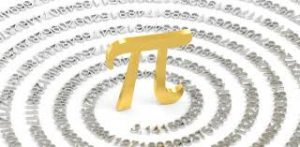first 17 letters in the Torah is 1776, their katan value is 42 and their middle letters sum to 756. And while that is the Pyramid’s base and the sum of the 6 faces of the Magical Essential Cube of 42, the 4 digits that precede it is …4206171776…., as in the hourglass (420). Just before He divided the Light from the darkness, He saw the Light and the Light was Good, tov (טוב) of numerical value 17.  The process is to eliminate the darkness, let it rise to the surface and burn it off.

The Light was good, tov (טוב) of numerical value 17. Hebrew is the interface to the Numbers, the Cosmic Letters of the universe and of the hyper-dimensions. Those Numbers are representations of forces, pressures, and fields. The Number 17 is the Prime Frequency of the Aether divided by or filtered through the Phi (φ) field, 27.5/φ = 17.  God saw the Light filtered (polarized) through the Phi (φ) field; it was good.  That is why it was good, because it was in harmony with the Cosmos.

There is a reason beyond the 13 colonies that the One (1) dollar bill has 13 stars, 13 arrows, 13 letters in E pluribus Unim, 13 layers of the pyramid with 72 bricks, 13 leaves, 13 stripes on the emblem, 13 berries, 13 letters in Annuit Coeptis, and 13 tiers in the cornucopia, even though by the time the first \$1 bills circulated there were far more than 13 states. Symbolism is everything.

### Darkness and Light

The full 4th verse of the Torah, “And God saw that the light was good; and God divided the light from the darkness (וַיַּרְא אֱלֹהִים אֶת הָאוֹר כִּי טוֹב וַיַּבְדֵּל אֱלֹהִים בֵּין הָאוֹר וּבֵין הַחשֶׁךְ)” has a numerical value of 1776 or42.014261502, as in the year 1776 HC when the darkness tried reuniting with the light by creating the Tower. There are many secrets in this verse, starting with its value using the Essential Cube Alef-bet cipher being 4248, the same as the standard value of the 22 Names of the 27 Letters, and then there is the 666 standard value of its 21 middle letters, or twice the value of “the darkness” 333, but it is the ratio between the incommensurate forces of darkness and Light that is most critical.

The numerical value of “the darkness” (333) less the numerical value of “the light” (212) is 121, as in 112, and the difference between their ordinal values is (45 32) = 13, Echad (One).

The Creator never said the darkness was bad, only that the Light was good and that the two needed to be separated for Creation to flourish. The darkness was already there—we are told as much in the Torah’s second verse—what G-d Created new was “the Light.” In the same way that G-d took a rib from Adam (45) and Created Chava (Eve), He took the Light out of the darkness, which is hinted at in the 45 letters and the 4500 albam gematria value of this verse.  This is the same 45 difference between the two hands of the Clockface (210165) = 45. In a similar manner the Creator separated the water from the water and placed a firmament between them to create and maintain the world above and the illusion below. Then the land was separated out of the water.

This in no way condones a proclivity for evil intentions and evil inclinations. The darkness was never declared good. The darkness is the illusion of physicality.

And like the darkness (333) and the Light (212), the day was split into “there was morning (333) and there was evening (303).” Together the phrase “there was morning (333) and there was evening (303)” = 636. This is the ratio 2/π or .636. Since this is the diameter (2/π) of a circle of circumference 2 we see that the 2 forces were already there, commingled in a circle of time, and the split was a separation. We see two separate clockfaces now, each measuring out 12 metaphysical hours.

The word for “the darkness (הַחשֶׁךְ)” appears only once within this verse, while “the Light (הָאוֹר)” appears twice.

# וַיַּרְא אֱלֹהִים אֶת הָאוֹר כִּי טוֹב וַיַּבְדֵּל אֱלֹהִים בֵּיןהָאוֹרוּבֵיןהַחשֶׁךְ

The two times “The Light” was repeated, each of numerical value 212, for a total of 424 is analogous to the two 21.2o gyrations and the 42.4o precession angles that the magnetic and dielectric fields are based on.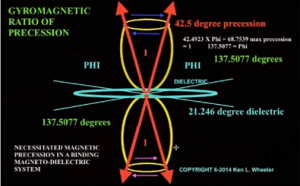### The Cosmic Harmonic

There can be no misunderstanding of the intentions of making their value equate to 424, the ultimate Moshiach Consciousness, nor of making their ratio be the Cosmic Harmonic, the Primordial Ratio 424/333 = 1.273.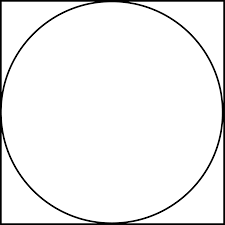This is the ratio of the area of the square to the inscribed circle, space to counterspace, as we just saw with the Clockface and as in the (Moon + Earth)/Earth ratio. One (1) and .273, the spiritual or universal consciousness component (1) and the physical or projected .273 to which all our physical elements conform.  This is the same 273 first born men that the Creator makes a point of counting separately in the Bamidbar (במדבר) and redeems each for 5 shekels. It is the same 273 that the Torah points out are above the 22,000 Levites in the total counting of the first-born men, 22,273.

The Creator built the ratio 1.273 into the Torah in the quantitative total of the 5 elements, 401273; and into the most basic measures of the Pyramid, 210/(330/2) = 1.273; and besides the (Moon + Earth)/Earth ratio (1.273); He built it into the ratio of the Sun/Earth (4 x 27.3); and the ratio of the Sun/(Earth and Moon) = 27.3π; and in 4/pi = 1.273…; and (4 – π)/π = 0.273….; and it all comes back to the simple geometric relationship of the 4-sided square to the inscribed circle (1.273).

The value of Moshiach Consciousness (424), the highest form of Cosmic Consciousness, is the square to the circle of darkness (333). The ratio is so precise that the value for darkness works out to 333.00 as in the 3330 years from the Torah’s reception in 2448 to the Event Horizon in 5778, the 66.6 jubilee years.

As a circle, Moshiach Consciousness (424) with a circumference of 424π equals (333 x 4.000), which not only refers to the 4 sides of a square and the darkness (333) but to Rav Ashlag’s equation or splitting 6000 years into .333 and .666 to get 2000 and 4000, as in the last 2000 years since the pivotal Divine Calendar year 3760 HC (0 CE), and then splitting it again two more times to end up with the 5778 Event Horizon of Consciousness.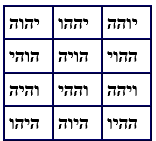Using the Cosmic Harmonic (1.273), when the Moshiach Consciousness (424) is the circle, the square becomes 541, Israel, whose current land area is 11,466 square miles, and each side of an actual square of  11,466 miles2 would be equal to 107.07 miles long, which is exactly 21√26 or the product of the higher Name of G-d, Ehyeh (אהיה) and the square root of the YHVH (יהוה). The land area of Israel is also exactly 90 sq miles, or (2 x 45) less than (2 x 5778), the 11,556 years Spherical Time diameter. The 90 miles to be sure is the sum of the 2 quintessential complementary Essential Cubes at the heart of it all, (42 + 48), the Magic Essential Cube of 42 and the Magic Essential Cube of the 48 Letters of the YHVH (יהוה).

More precisely, when the gyromagnetic precession (42.489) and/or the Torah’s 4th verse about dividing the Light (4248) and/or the 22 Names of the Letters (4248) is the circle, the square becomes 541, Israel.

The same Cosmic Harmonic (1.273) when applied to 378 is 481.25, the height of the Tower of Truth, the Great Pyramid, and the Hand of the Clockface in feet.  This applies whether it is the 378 total ordinal value of the Alef-bet or of the Essential Cube of Creation, and/or of the 378-value of “Sinai Desert Bamidbar Sinai (במדבר־סיני),” and/or to the complete value of the Hebrew word “HaChoshech (הַחשֶׁךְ), the darkness” that is (333 + 45) = 378.When applied to the Essential Cube of Creation it is the Hand pointing straight up and to the darkness it is the Hand pointing down. Those two opposing positions of the Hands of Time within the Clockface also refer to the two mirrored Essential Cubes, referring to the Binah one as the Light and the Zeir Anpin/Malchut Essential Cube as the darkness because it creates physicality, or at least the illusion of it. The Tower to Binah is the square to the circle of darkness, physicality.We know well the relationship between Phi (φ) and the Primal Frequency 27.5 Hz, so it is interesting that when the Cosmic Harmonic (1.273) is applied to it, in other words when 27.5 Hz becomes the circle, the square has a total area of 35.00 as in the doubled 35th verse. Likewise, the square of the gateway’s 55’ circle has a total square area of 70.00, as in the two 35 year periods surrounding the center of David’s 70 year life in the middle of the Spherical Time radius, etc.

We also see that the Phi (φ) angle (137.5o) as the circle becomes the 175.0 years of Abraham’s life as the square.

While the Pyramid’s 35th course is markedly of double height, the 35th cycle of 137.5o is 4812.5 or exactly (10 x 481.25’), the height of the Pyramid in feet, and the 42nd cycle of 137.5o is the 210th of 27.5o or a total of 5775o, representing the height in cubits (210) and inches 5775”.  This is the first interval between the Singularity (42), the Primal Frequency (27.5 Hz), and the Phi (φ) angle (137.5o) of rotation. It is the Hand of Time.

### The Washington MonumentThere are 3 independent city-states in the world, the Vatican, the City of London, and the District of Columbia in the area formerly known as Rome on the Potomac. All 3 have giantEgyptian obelisks in strategic positions.

The ratio of the Essential Cube to the Tower of Truth is 210/378 = .555 just like the ratio of the area of the Pyramid’s base to its Capstone base, (3302/142) = 555. It is also just like the towering Washington Monument that was built 555’ high and 55.5‘ wide at the base and is situated across 14th Street from the 541-room Capitol Building, as in Israel (541) and as in (541 + 14) = 555. Both the 48.1 story Tower of Truth and the 55.5 story obelisk called the Washington Monument are built on a similar 1:10 proportion.  The masons who built the Washington Monument and the Capitol Building and who dedicated the 22 foundation stones of the monument must have had some inside knowledge considering the Tower of Truth was hidden under the 2.3 million stone blocks of the encasing Pyramid.

Of course, that makes the Washington Monument 666” wide at the base and 6660” tall, but its just symbolic.  And the Great Pyramid Capstone base to the Washington Monument base ratio is 32.083’/55.5’ = .5780 but how would they know anything about the 14 cu wide base of the Capstone?

# The Sphere of One

### The Great Pyramid as a Sphere.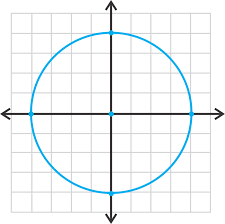The dimensions of the Great Pyramid can be plotted as a sphere in 3-dimensions, and they interact with the coordinates of Pi (π), Phi (φ) and the .666 proportion.

Like the Pyramid Clockface and Spherical Time, a circle has a simple formula that determines its circumference by virtue of the length of its radius. The radius of the circle is the distance from the center point of the disk to its boundary or outer edge, the ring or circle itself. That formula is.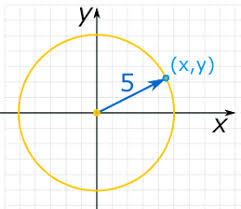We might think that any point on the circle is like any other, and by definition they do all share the exact same radius, yet as it turns out, each point is extremely unique, and in the case of Spherical Time it is defined by Phi (φ) and the rate of rotation. If we place the circle in the center of an (X,Y) graph with (0,0) as the central point, every point on the circle will have a different (X,Y) set of coordinates.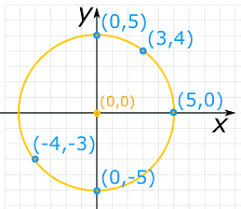Those coordinates will be solely determined by the length of the radius and the Pythagorean theorem: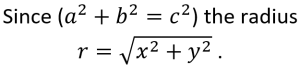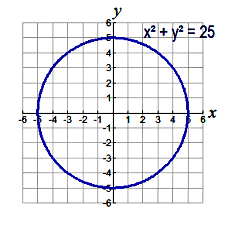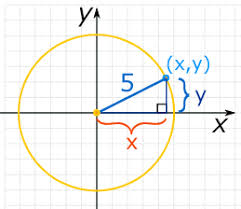This holds true for 3-dimensional spheres, and any-dimensional sphere for that matter. The general formula is: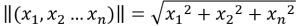When we assign a radius of 1(One) to the circle, the formula for determining the (X,Y) set of coordinates is: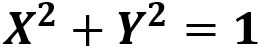Using this formula, we see that the base of the Pyramid in inches, 9075 and the number 42.000 are complementary coordinates on the Circle of One (1).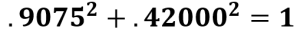Since a 3-dimensional sphere has a third axis (Z), the equation for a sphere is: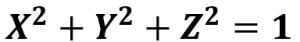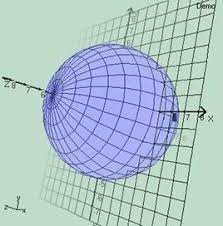It seems like a complicated way of viewing a simple circle or a simple sphere such as a ball. Nevertheless, these equations are a very important first step for our understanding of the higher dimensions, such as the individual and unique locations of the time-paths.

Numbers can be visualized as specific points in space and as such, they have coordinates on the X, Y and Z axis, positions in a network that in the 3rd dimension of physical space form a sphere. These are points in a matrix, yet they are bound by the surface shape of the sphere, or the circumference of a circle that is fixed in position. They are no longer floating freely in space, though the sphere they are in can be.  We are limiting ourselves here to 3 dimensions, but the process is the same for our 8-dimensional and 248 dimensional models as well. The point is that our recurrent Torah, Cosmic, and Pyramid numbers may not be simply numbers but grid points in multiple dimensions.

Since we are working with circles and spheres of radius 1, all the coordinates need to be under 1 and so all the numbers need to be reduced to a decimal. For example, 756.25 ft would be represented as .75625.

For starters, using Pi (π) and 1/Phi(φ) as the X and Y coordinates, the Z axis coordinate is 72: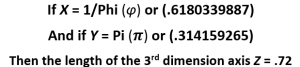This equation has profound implications for the Great Pyramid because 1/φ2 is the descending slope angle of the Pyramid and .722 is its ascending slope angle, meaning the coordinates of a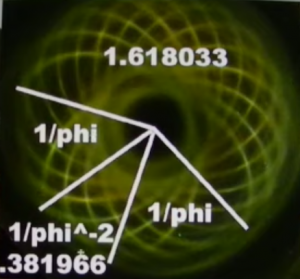sphere of radius One (1) that utilize Pi (π) as a 3rd coordinate is what defines the Pyramid’s shape and dimensions. Moreover, the 2 coordinates squared π2 and 1/φ2 together equal approximately .481, the height of the Pyramid, while π2 and (1/φ2)2 equal approximately 10.0.

Yet another defining example using a sphere of radius One (1) is found by balancing the coordinates of the YHVH (יהוה) with the Singularity of 42, that also represents the Pyramid’s 42o edge angle to get the base of the Pyramid.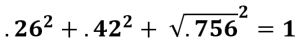And since 756 is the Primal Frequency (27.5”) squared, the Primal Frequency is the actual 3rd spatial coordinate to the YHVH (יהוה) and the Source.

When we insert the Primal Frequency (27.5”) as a coordinate, we get both the base (756.25’) and the height (481.25’) of the Pyramid along with the .666 proportion as the balancing coordinate.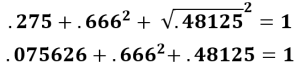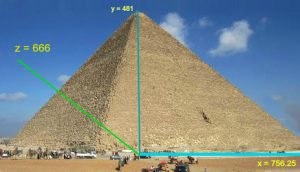One of things we should keep in mind is that all of these coordinate values are individual vectors within an array, like the 26 vectors in the Essential Cube of Creation whose total distance for the sum of the 26 squares and 26 cubes is (1638 + 16562) = 18200, as in the 1820 YHVH (יהוה) in the Torah, the 182 doubled words in the Torah, and Jacob (182).

Another connection between the Pyramid and the Sphere of One (1) is found in the sum of is height (210 cu) and base (756’) and keter (620):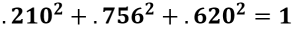And yet another is found with the semi-diagonals (233.33 cu) and/or casing stone measurement (233.33”) with the Pyramid’s base (756.25’) and its Apothem (611.81’)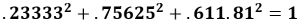Likewise, the two scored lines in the Entrance Passage combine with the Pyramid’s Apothem to lie on the Sphere of One (1).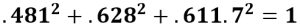Considering that 722 is the slope angle (51.84o) it is only fitting that it too fit perfectly into the Sphere of One (1) schematic, alongside the Cosmic Consciousness, Moshiach Ben David (424) and the Pyramid’s Entrance height (55’):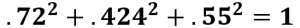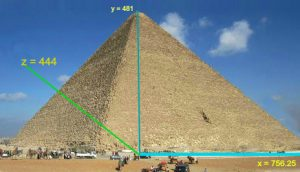The Pyramid was morphed from a giant sphere whose radius is 1. Nevertheless, those numbers will not scale together, but these coordinates will when the .444 ratio is incorporated: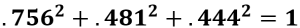### The Sphere of Time

Seeing as 444 relates to the year 2018 and thus to 5778, as explained by Rav Ashlag, when we use those dates in the Spherical Time sphere as coordinates, their balancing coordinate is the square root of .625424, as in H’Keter and Moshiach Ben David, the highest level of the Cosmic Consciousness.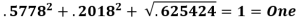And while not points on this sphere, the two parallel timeframes, Spiritual (3760) and Physical (2018) work out to .57782 + .20182 = .1820, as in the 1820 YHVH (יהוה) in the Torah and the Path of One.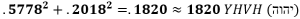It was all designed from the start, even before the perception of existence. The matrix is geometric as is everything in it, including time. The only way this is possible is in a Divine Simulation.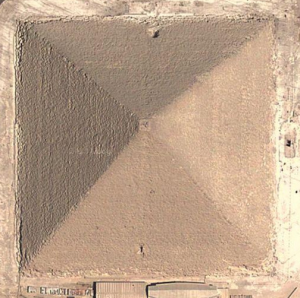Envisioning a 4-dimensional sphere whose coordinates are the 4 Essential Elements of Creation, the 4 sets of the 112 Triplets that are woven into the Torah and the Great Pyramid in myriad ways, we see that they have a radius of (.91432 + .37012 + .11182 + .30032) = √1.0756, as in the 107th Triangular Number and the base of the 4-sided Pyramid.

Moreover, their sum 16,965 is equivalent to the height and base of the Pyramid in all 3 measuring units plus 336 for their 336 letters.

Is this coincidence or do the higher-dimensional networks of the Essential Triplets impact the design of Spherical Time and the schematics of the Great or Cosmic Pyramid?

As we said earlier, the Great Pyramid and most of the giant pyramids were designed and built with a functional purpose and not for selling camel rides.

Like the 112 Essential Triplets, the Unified Names of the Creator that sum to 112, were known to the ancient Kabbalists as Yabok (יבק) of numerical value 112. Spelled-out Yabok (יוד־בית־קוף) has the value 618 or 1/Phi(φ). The deeper we dig, the more functional the universe becomes. Spelling it out further, all 3 iterations including the milui of the milui (יוד־ויו־דלת־בית־יוד־תו־קוף־ויו־פא) equal (112 + 618 + 1603) = 2333, as in the semi-diagonals (233.33 cu) and/or casing stone measurement (233.33”) and the 23.33 cu difference of the diagonals within the Pyramid’s base. This is like the 8 corners of the Essential Cube that add up to 112. Designed functionality!

While there appear to be a plethora of connections between the coordinates of the Sphere of One (1) and the Great Pyramid and/or Spherical Time, connections to significant numbers for all 3 coordinates are exceedingly rare.  If we were to unwrap that Sphere of One (1) and lay it flat like a map, it appears we would find a grid or network that connects all the major dimensions of the Great Pyramid, and intersects them with a grid of Spherical Time. It is an unbelievable design—one that could only be conceived in hyper-dimensional dynamics!

When we add the 70 letters in the next level or iteration to Yabok (יבק)to the 37 in the first three levels, the total is 107 letters that equal 6181 or 1/Phi(φ) again, integrated into the event Horizon.

One Biblical combination that does work is Abraham (808) and Sarah (505) with the 3rd coordinate being 303, as in 358, Moshiach.  This is a very special case indeed as (808505) = 303 and (.8082 + .5052 + .3032) = 1. As you will recall, both their Names were specifically altered and given to them by the Creator, as they founded the religion and philosophy of the One (1), just as (808 + 505) = 1313, the seed of the Alef-bet and date (1313 BCE) of the Torah reception at Sinai.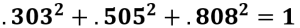### The Sphere of Consciousness

Another such significant combination is the square root of 42 or 6480 paired with the value of the 42-Letter Name, 3701. Their 3rd coordinate on the Sphere of One (1) is 6666, as in the 42 letters of the Sword of Moses, meaning this Cosmic equation holds 3 different representations of 42.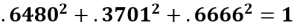Meanwhile, the value of the 42-Letter Name, 3701, paired with the Torah’s first verse associated with that Name has a 3rd coordinate on the Sphere of One (1) of 8888.Meanwhile, at the outset of this paper, we pointed out the relationship to Egypt between the 4805 occurrences of the letter Pe (פ) in the Torah, out of the total 304805 letters, and that they have a total numerical value of (80 x 4805) = 384,400, which not only aligns them with the 384,400 kilometer distance between the Earth and the Moon, but with Keter, as it is exactly 6202.  As it turns out 4805 is also on the Sphere of One and its complementary coordinates are 620 and 620, as in 6202. Keep in mind, proper combinations to significant numbers are exceedingly rare, and that all these delineated combinations lie on the surface of the same dynamic Sphere of One (1).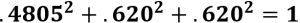Now since the radius of our Spherical Time bubble is 5778 years, we should see which vectors or coordinates get us to the Event Horizon surface of the bubble. As it turns out, the resulting vectors are 363 and 363, as in HMoshiach, the Cosmic Consciousness, and √3142 as in so many of the Names of G-d, Pi (π), and the perimeter of the central vertical plane of the Pyramid divided by the Primal Frequency, (267 + 267 + 330)/27.5 = 314.2 cu.

The 2 vectors 363 are more precisely 36304.24470 as in the Spherical Time circumference (36304.24470), and √3142.00363.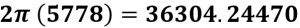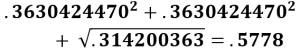The secret is in the consciousness and in the Names. There are two ways to burst the bubble. One is to let time expire. It that case everything resets, and our journey goes back to square one. The other is to solve the puzzle and consciously connect to the Names in the proper way.  This is the way of Enlightenment, the Path of Wisdom, and the Path of the Tree-of-Life.